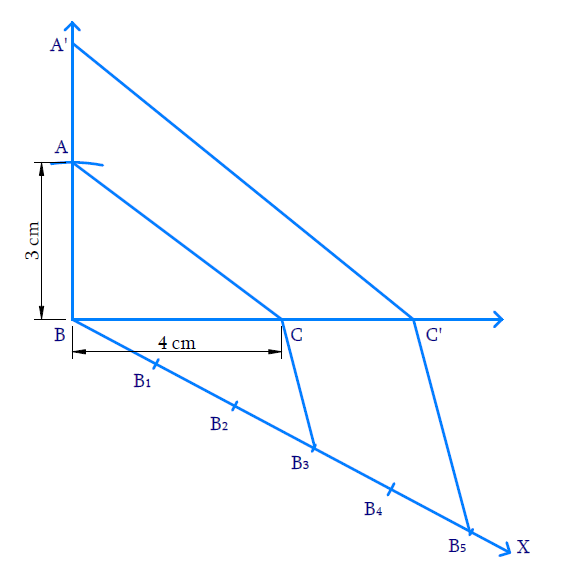# Ex.11.1 Q7 Constructions Solution - NCERT Maths Class 10

Go back to  'Ex.11.1'

## Question

Draw a right triangle in which the sides (other than hypotenuse) are of lengths $$4 \,\rm{cm}$$ and $$3 \,\rm{cm}$$. Then construct another triangle whose sides are \begin{align}\frac{5}{3}\end{align} times the corresponding sides of the given triangle.

Video Solution
Constructions
Ex 11.1 | Question 7

## Text Solution

What is known?

$$2$$ sides and the angle between them and the ratio of corresponding sides of $$2$$ triangles.

What is unknown?

Construction.

Reasoning:

• Draw the triangle with the given conditions.
• Then draw another line which makes an acute angle with the base line. Divide the line into $$m + n$$ parts where $$m$$ and $$n$$ are the ratio given.
• Two triangles are said to be similar if their corresponding angles are equal. They are said to satisfy Angle-Angle-Angle (AAA) Axiom.
• Basic proportionality theorem states that, “If a straight line is drawn parallel to a side of a triangle, then it divides the other two sides proportionally".

Steps:Steps of constructions:

(i) Draw $${{BC = 4\, \rm{cm}}}{{.}}$$ At  $$B,$$ make an angle $$\angle {{CBY}} = {90^ \circ }$$ and mark $$A$$ on $$BY$$ such that $${{BA = 3 }}\,{\rm{cm}}{{.}}$$ Join $$A$$ to $$C.$$ Thus $${{\Delta ABC}}$$ is constructed.

(ii) Draw the ray $$BX$$ so that $$\angle {{CBX}}$$ is acute.

(iii) Mark $$5$$ ($$5 > 3$$ in $$\frac{5}{3}$$ ) points $${{{B}}_{{I}}}{{,}}\,{{{B}}_{{2}}}{{,}}\,{{{B}}_{{3}}}{{,}}\,{{{B}}_{{4}}}{{,}}{{{B}}_{{5}}}$$ on $$BX$$ so that

\begin{align}&{{B}}{{{B}}_{{1}}}\\=&{{{B}}_{{1}}}{{{B}}_{{2}}}\\=&{{{B}}_{{2}}}{{{B}}_{{3}}}\\=&{{{B}}_{{3}}}{{{B}}_{{4}}}\\=&{{{B}}_{{4}}}{{{B}}_{{5}}}\end{align}

(iv) Join$${B}_{3}$$ ($$3^\rm{rd}$$ point on $$BX$$ as $$3<5$$) to $$C$$ and draw $${{{B}}_{{5}}}{{C'}}$$ parallel to $${{{B}}_{{3}}}{{C}}$$ so that $${{C'}}$$ lies on the extension of $$BC.$$

(v) Draw $${{C'A'}}$$ parallel to $$CA$$ to intersect of the extension of $$BA$$ at $$A’.$$

Now $${{\Delta BA'C'}}$$ is the required triangle similar to $${{\Delta BAC}}$$ where

\begin{align}\frac{{{{BA'}}}}{{{{BA}}}}{{ = }}\frac{{{{BC'}}}}{{{{BC}}}}{{ = }}\frac{{{{C'A'}}}}{{{{CA}}}}{{ = }}\frac{{{5}}}{{{3}}}\end{align}

Proof:

In $${{\Delta B}}{{{B}}_{{3}}}{{C',}}\,\,{{{B}}_{{3}}}{{C}}\,\,{{||}}\,\,{{{B}}_{{3}}}{{{C}}^{{1}}}$$

Hence by Basic proportionality theorem,

\begin{align} \frac{{{{B}}_{{3}}}{{{B}}_{{5}}}}{{B}{{{B}}_{{3}}}}&{=}\frac{{CC }\!\!'\!\!{ }}{{BC}}{=}\frac{{2}}{{3}} \\ \frac{{CC }\!\!'\!\!{ }}{{BC}} {+1}&=\frac{{2}}{{3}}{+1}\qquad \text{(Adding}\,\,{1)} \\ \frac{{CC }\!\!'\!\!{ +BC}}{{BC}} &{=}\frac{{5}}{{3}} \\ \frac{{BC }\!\!'\!\!{ }}{{BC}}&{=}\frac{{5}}{{3}} \end{align}

Consider $${{\Delta BAC}}$$ and $${{\Delta BA'C'}}$$

$$\angle {{ABC}} = \angle {{A'BC'}} = 90^\circ$$

$$\angle {{BCA}} = \angle {{BC'A'}}$$ (Corresponding angles as $${{CA}}\,\,\,{{||}}\,\,{{C'A'}}$$ )

$$\angle {{BAC}} = \angle {{BA'C'}}$$

By AAA axiom, $${{\Delta BAC}}\,\sim\,{{\Delta BA'C'}}$$

Therefore corresponding sides are proportional,

Hence,

\begin{align}\frac{{{{BA'}}}}{{{{BA}}}}{{ = }}\frac{{{{BC'}}}}{{{{BC}}}}&{{ = }}\frac{{{{C'A'}}}}{{{{CA}}}}\\&{{ = }}\frac{{{5}}}{{{3}}}\end{align}

Learn from the best math teachers and top your exams

• Live one on one classroom and doubt clearing
• Practice worksheets in and after class for conceptual clarity
• Personalized curriculum to keep up with school